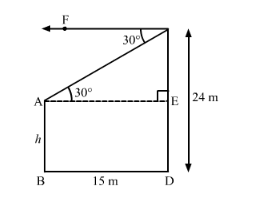# The horizontal distance between two poles is 15 m.

Question:

The horizontal distance between two poles is 15 m. The angle of depression of the top of the first pole as seen from the top of the second pole is 30°. If the height of the second pole is 24 m, find the height of the first pole. $(\sqrt{3}=1.732)$

Solution:Let AB be the first pole and CD be the second pole.

Distance between the two poles, BD = 15 m

Height of the second pole, CD = 24 m

Suppose the height of the first pole be h m.

Draw AE ⊥ CD.

∴ CE = CD − ED = (24 − h) m                       [AB = ED = h m]

AE = BD = 15 m

Now, $\angle \mathrm{CAE}=\angle \mathrm{ACF}=30^{\circ}$ (Alternate angles)

In right $\triangle \mathrm{ACE}$,

$\tan 30^{\circ}=\frac{\mathrm{CE}}{\mathrm{AE}}$

$\Rightarrow \frac{1}{\sqrt{3}}=\frac{24-h}{15}$

$\Rightarrow \frac{15}{\sqrt{3}}=24-h$

$\Rightarrow h=24-5 \sqrt{3}$

$\Rightarrow h=24-5 \times 1.732=15.34 \mathrm{~m}$

Hence, the height of the first pole is 15.34 m.